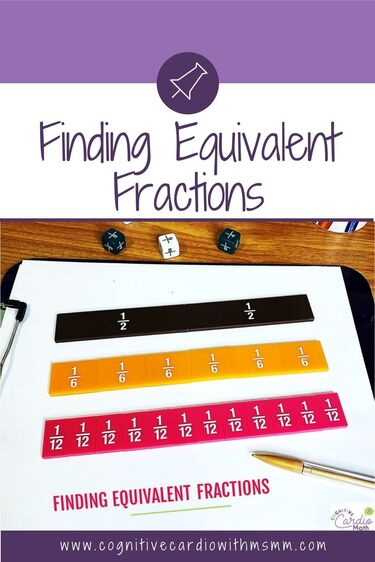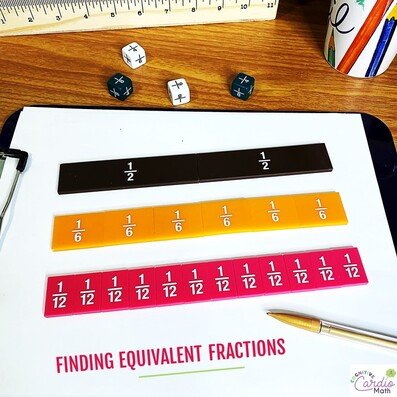# Teaching Fractions in Middle School Math: Finding Equivalent FractionsFraction Series, Week 4: Equivalent Fractions

We talked a little bit about equivalent fractions in the Week 2, modeling post, with the idea of using models, or representations, when adding or subtracting fractions, but we didn’t talk about how to find equivalent fractions, so we’ll go into that here in this quick post.

First off, when do we usually use equivalent fractions?
We often use equivalent fractions when:

• comparing fractions
• dividing fractions
• we can even use them when multiplying fractions, though it just creates more work:-)

​Once we decide we need equivalent fractions, we find a common denominator for the fractions.  There are a few ways to do that:

• Multiply the denominators together (not a personal favorite)
• List the multiples of the denominators to find the least common multiple/least common denominator
• Use prime factorization

Let’s look at each one, using the same fractions each time, just for comparison’s sake. We could be adding these, comparing these, or dividing these…..it doesn’t really matter; but we’ll choose adding for these examples

Method 1: Let’s start with multiplying the denominators together, 12 x 8. This gives us a common denominator of 96.
Then students must multiply 5/12 by 8/8 to get 40/96 and 7/8 by 12/12 to get 84/96.
The addition and simplifying are show in the image  below.
Using this method does seem pretty easy for students who have trouble finding the LCM, but it ends up giving them larger numbers (124/96) to simplify.

• There’s a good chance that those same students having trouble finding LCM would have difficulty simplifying the larger numbers in their answer.
Method 2: Find LCM/LCD by listing the multiples.
One benefit of using this method is that it helps students practice their multiplication facts. BUT, if students don’t know the multiplication facts, it might take a while to create the lists (they could use a multiplication chart to help them).
​The multiple lists of 8 and 12 are below.
In the lists we can see that 24 is the LCM/LCD. To help students remember what to multiply each fraction by to get their equivalent fractions, I’ve found it can help to count which multiple the LCM is in each list.

• For example, in the list of 8’s multiples, 24 is the 3rd multiple (8 x 3 is 24), so we’d multiply 7/8 by 3/3.
• 24 is the 2nd multiple in the 12’s list (12 x 2 = 24), so we’d multiply 5/12 by 2/2.
Using the LCM keeps the numbers in the fractions smaller, and in this case, gives us an answer that’s easier to simplify – students just need to convert from an improper fraction (fraction greater than one) to a mixed number.
Method 3: Find LCM/LCD using prime factorization
Students can use a factor tree or the ladder method to find the prime factorizations.

Benefits of this method:

• Students can see which prime factors the numbers have in common
•
• Students can see how both the common and ‘uncommon’ factors contribute to the LCM.

The prime factorizations for 12 and 8 are:
12: 2 • 2 • 3
8: 2 • 2 • 2

To find the LCD/LCM:
​   1) Identify the factors that 12 and 8 have in common (two 2s).

2) Identify the factors they DON’T have in common (another 2 and a 3).
3) Multiply the common factors by the ‘uncommon’ factors (three 2s and a 3).

LCD/LCM: 2 • 2 • 2 • 3 = 24
4) To help students decide what to multiply each fraction by to get their equivalent fractions (other than asking “what do you multiply 12 by to get 24”), students identify which factor ISN’T in each number’s prime factor list. In this case, 3 isn’t in the list of factors for 8, so 7/8 is multiplied by 3/3.
The third 2 isn’t in the factor list of 12, so 5/12 is multiplied by 2/2.
And we end up with the same equivalent fractions as above (10/24 and 21/24).
Method 4: Find the LCM/LCD using the ladder method
To use the ladder method for LCD, we put both denominators into the ladder, side-by side, as shown in the diagram below.

• We identify a prime factor and then divide by that factor
• We identify a 2nd prime factor in the resulting quotients and divide again
• We continue identify and dividing by prime factors, until there are no common factors other than 1

(For a more detailed explanation about how the ladder method works, check out the posts listed below.)

Once we’ve removed all the common factors, we get the LCM/LCD by multiplying all the numbers on the outside of the ladder.

• LCM/LCD: 2  2  2  3 = 24

To find the equivalent fractions, students use the factor at the bottom of the ladder that’s under the opposite number

• In this case, 3 is under 12 and isn’t a factor of 8, so 7/8 is multiplied by 3/3.
• 2 is under 8 and is an ‘extra’ 2 that’s not included in the factors of 12; so 5/12 is multiplied by 2/2.

Using the Ladder Method in Middle School Math, for GCF, LCM, Factoring

Using Representations for Equivalent FractionsWhatever method you use, incorporating models will help students understand that the equivalent fractions represent the same amount. (This is a bit easier when the fractions use smaller numbers.)

In the image, for example, we might be adding 1/2 and 5/6.

• When we convert 1/2 to 3/6, the representation using the fraction strips helps students see that these fractions represent the same amount.

Or, if we were comparing 5/6 and 11/12, we could use the fraction strips to verify that 5/6 is equivalent to 10/12.

Which method do you prefer for finding LCD and equivalent fractions?
Do you use fraction strips or another method to model the equivalences?

Interested in more fraction and fraction operation content?
Check out the course,

## EllieWelcome to Cognitive Cardio Math! I’m Ellie, a wife, mom, grandma, and dog ‘mom,’ and I’ve spent just about my whole life in school! With nearly 30 years in education, I’ve taught:

• All subject areas in 4th and 5th grades
• Math, ELA, and science in 6th grade (middle school)

I’ve been creating resources for teachers since 2012 and have worked in the elearning industry for about five years as well!